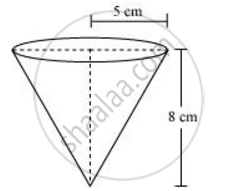Share

# A vessel is in the form of an inverted cone. Its height is 8 cm and the radius of its top, which is open, is 5 cm. It is filled with water up to the brim. When lead shots, each of which is a sphere of radius 0.5 cm are dropped into the vessel, one-fourth of the water flows out. Find the number of lead shots dropped in the vessel. - CBSE Class 10 - Mathematics

ConceptVolume of a Combination of Solids

#### Question

A vessel is in the form of an inverted cone. Its height is 8 cm and the radius of its top, which is open, is 5 cm. It is filled with water up to the brim. When lead shots, each of which is a sphere of radius 0.5 cm are dropped into the vessel, one-fourth of the water flows out. Find the number of lead shots dropped in the vessel.

#### SolutionHeight (h) of conical vessel = 8 cm

Radius (r1) of conical vessel = 5 cm

Let n number of lead shots were dropped in the vessel.

Volume of water spilled = Volume of dropped lead shots

1/4 xx "Volume of cone" = n xx 4/3 r_2^3

1/4xx1/3pir_1^2h = n xx 4/3pir_2^3

r_1^2h = n xx 16r_2^3

5^2xx8 = nxx 16xx (0.5)^3

n = (25xx8)/(16xx(1/2)^3) = 100

Hence, the number of lead shots dropped in the vessel is 100.

Is there an error in this question or solution?
Solution A vessel is in the form of an inverted cone. Its height is 8 cm and the radius of its top, which is open, is 5 cm. It is filled with water up to the brim. When lead shots, each of which is a sphere of radius 0.5 cm are dropped into the vessel, one-fourth of the water flows out. Find the number of lead shots dropped in the vessel. Concept: Volume of a Combination of Solids.
S